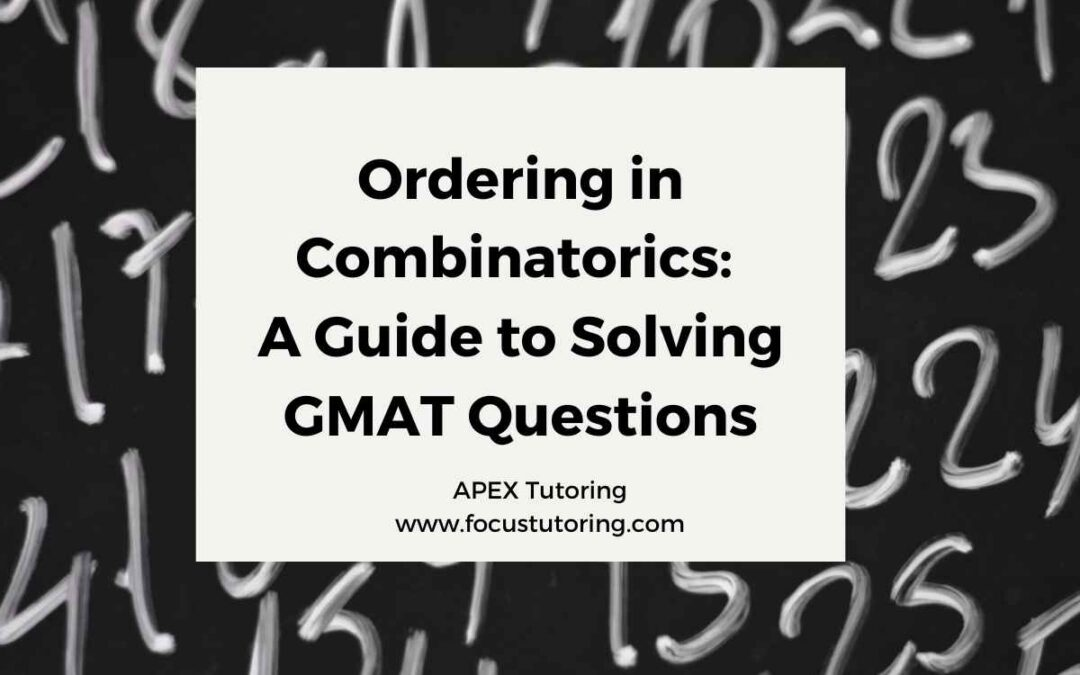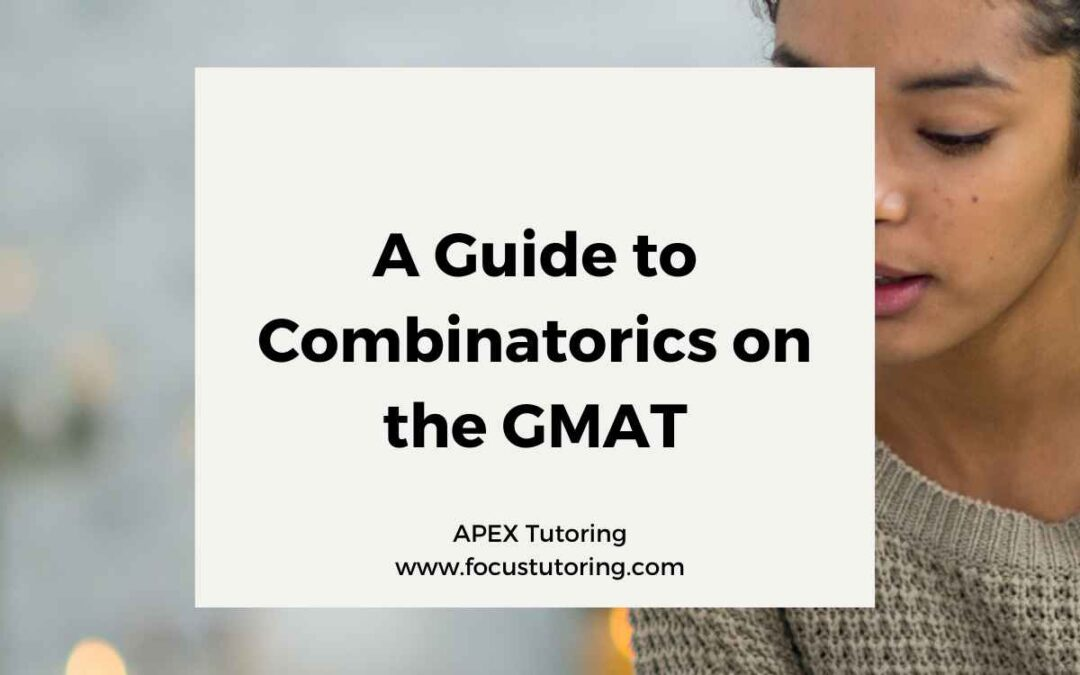## How to Solve Permutations Questions on the GMAT

Permutations are a type of combinatorics task that is often tested on the GMAT. In a permutations question, you are asked to find the number of ways to order a group of elements. The order of the elements matters in permutations questions, so the number of possible...## Ordering in Combinatorics: A Guide to Solving GMAT Questions

What is an Ordering Task? Ordering tasks are a common type of question on the GMAT. In an ordering task, you are asked to determine the number of ways to order a set of objects. The number of ways to order n different objects is n!, or n factorial. The best way to...## A Guide to Combinatorics on the GMAT

Every combinatorics question on the GMAT involves one or more of three fundamental combinatorics tasks: ordering, permutations, and combinations. This article will introduce the three tasks, and each of the three following articles will explain a task intuitively to...## The Basics of GMAT Combinatorics

By: Apex GMAT Contributor: Svetozara Saykova Date: 24th June 2021 Combinatorics can seem like one of the most difficult types of questions to come across on the GMAT. Luckily there are not many of them within the exam. Still these questions make up the top level of...## When Probability Meets Combinatorics: One Problem, Two Approaches

By: Rich Zwelling, Apex GMAT Instructor Date: 16th March, 2021 Now, we’d like to take a look at an Official GMAT Probability problem to pull everything together. The following is a good example for two reasons:  1. It illustrates a quirky case that is difficult more...